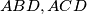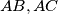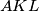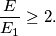### IMO Shortlist 1988 problem 13

Kvaliteta:
Avg: 0,0
Težina:
Avg: 0,0
In a right-angled triangle$ABC$ let$AD$ be the altitude drawn to the hypotenuse and let the straight line joining the incentres of the triangles$ABD, ACD$ intersect the sides$AB, AC$ at the points$K,L$ respectively. If$E$ and$E_1$ dnote the areas of triangles$ABC$ and$AKL$ respectively, show thatIzvor: Međunarodna matematička olimpijada, shortlist 1988